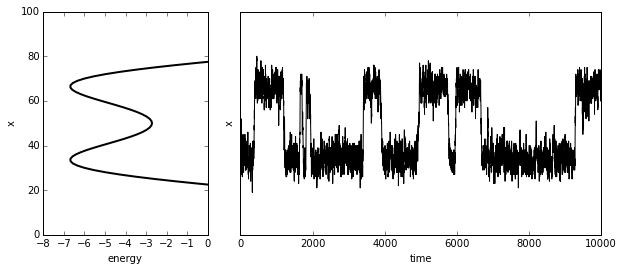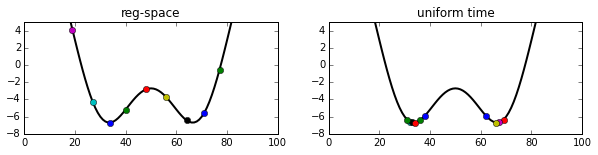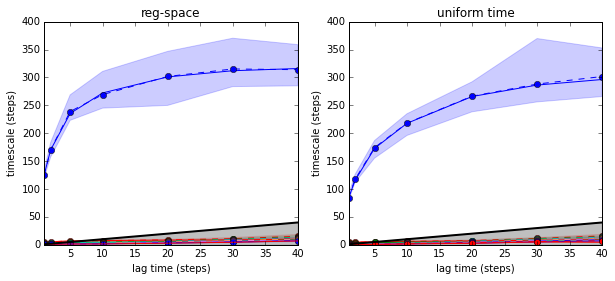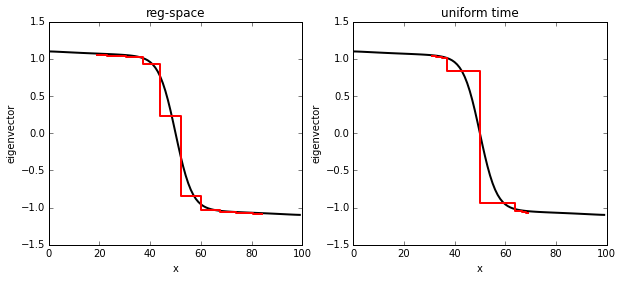# Regular space clustering¶

 Summary: Regular space clustering is a simple and fast clustering algorithm that attempts to distribute cluster centers approximately uniformly in space, and then employs a Voronoi discretization to partition the space. This is an efficient way of clustering data for Markov model generation especially when the time-lagged independent component analysis (TICA) has been used to produce coordinates.

Regular space clustering is a fast and simple clustering method that has been suggested in , and is summarized in Algorithm 1. Regular space clustering is very similar to Hartigan’s Leader algorithm

## Algorithm¶

 Algorithm 1: Regspace clustering Input: Data $\mathbf{X}=(x_{1},\,...,\, x_{T})$, distance metric $d(x,y)$, distance threshold $d_{\mathrm{min}}$ Output: Cluster centers $\mathbf{Y}=(y_{1},\,...,\, y_{n})$, discretized data $(s_{1},\,...,\, s_{T})$ 1. $y_{1}:=x_{1}$, $n:=1$ 2. For $t=2,...,T$: If $\min_{i=1}^{k}d(x_{t},y_{i})>d_{\mathrm{min}}$: 2.1. $y_{n+1}:=x_{t}$ 2.2. $n:=n+1$ 3. Return $\mathbf{Y}=(y_{1},\,...,\, y_{n})$
Example

Fig. 1 shows that for all clustering methods and numbers of clusters (10, 100, or 1000) used, the slowest implied timescales converge to approximately the same values $t_{2}\approx25$ ns and $t_{3}\approx10$ ns at long lag times $\tau$. All clustering methods produce MSMs which converge for smaller values of $\tau$ when increasingly many clusters are used. This tendency can be assumed as long as sufficient statistics are available. When the number of clusters gets too large for a given amount of simulation data, statistical issues need to be considered. The differences in MSM quality between the different clustering methods for similar numbers of clusters are small. Interestingly, $k$-centers and regular space clustering do not outperform the simple method of picking cluster centers at regular time intervals. The three methods used here are relatively fast, all having a time complexity of $O(kN)$, with $k$ being the number of clusters and $N$ the number of frames in the trajectory. It is unclear whether using computationally more expensive clustering methods are able to significantly benefit the MSM construction at this stage. Our findings suggest that MSM construction from trajectory data is robust as long as sufficient data is available and a sufficient number of states are used.Figure 1: Lag time dependent implied timescales $t_{2}$ (solid lines) and $t_{3}$ (dashed lines) of the slowest processes computed from Markov models of MD simulation data of the MR121-GSGSW peptide. a) regular space clustering. b) cluster centers chosen from frames at fixed time intervals.

#### Citing regular space clustering:¶

The algorithm was suggested in .

## Assessing the performance of regular space clustering on a double-well potential¶

We first load all packages used here

In :
# numerics
import numpy as np
# emma
import pyemma.coordinates as coor
import pyemma.msm as msm
import pyemma.msm.io as msmio
# plotting
import pyemma.plots as emmaplt
%pylab inline

Populating the interactive namespace from numpy and matplotlib


We load the double-well energy function and a trajectory generated using a diffusive dynamics on it:

In :
E = np.loadtxt('./data/2well_energy.dat')


Plot the energy function and the first chunk of the trajectory, to illustrate the metastability

In :
figure(figsize=(10,4))
ax1 = subplot2grid((1,3),(0,0))
ax1.plot(E, range(0,len(E)), color='black', linewidth=2)
xlim(-8,0); xlabel('energy'); ylabel('x')
ax2 = subplot2grid((1,3),(0,1),colspan=2)
ax2.plot(X[:10000], color='black')
ylim(0,100); xlabel('time'); ylabel('x'); yticks([])

Out:
([], <a list of 0 Text yticklabel objects>)Now we cluster the trajectory data with regular space clustering and uniform time clustering, in order to contrast two different methods

In :
# regular space clustering
cl_space = coor.cluster_regspace(data=X, dmin = 5)
# sort cluster centers by x-coordinate (for visualization)
centers_space = np.sort(cl_space.clustercenters, axis=0)
# uniform time clustering
cl_time = coor.cluster_uniform_time(data=X, k=10)
# sort cluster centers by x-coordinate (for visualization)
centers_time = np.sort(cl_time.clustercenters, axis=0)

2015-04-19 16:26:16,722 RegularSpaceClustering[0x1087f4b50] INFO     Running regular space clustering
2015-04-19 16:26:16,728 UniformTimeClustering[0x1087f4d50] INFO     Running uniform time clustering


Both methods have produced 10 cluster centers. We plot them into the energy function

In :
# plot regular space cluster centers
figure(figsize=(10,2))
ax1 = subplot2grid((1,2),(0,0))
ax1.plot(E, linewidth=2, color='black')
for c in cl_space.clustercenters:
ax1.plot([c], [E[int(c)]], marker='o')
ylim(-8,5); title('reg-space')
# plot uniform time cluster centers
ax2 = subplot2grid((1,2),(0,1))
ax2.plot(E, linewidth=2, color='black')
for c in cl_time.clustercenters:
ax2.plot([c], [E[int(c)]], marker='o')
ylim(-8,5);  title('uniform time')

Out:
<matplotlib.text.Text at 0x10a0201d0>It is apparent that the regular space clustering has produce approximately uniformly distributed cluster centers, while the uniform time clustering puts all the centers close to the energy minima, where nearly all of the stationary probability is.

We now discretize the trajectory to either set of cluster centers

In :
Sspace = coor.assign_to_centers(X, centers_space)
Stime  = coor.assign_to_centers(X, centers_time)


In order to compare the quality of these two clusterings, we construct Markov state models at a series of lagtimes and compute the slowest relaxation timescale.

In addition to the maximum likelihood estimates, we also compute a bootstrap error estimate

In :
# Compute relaxation timescales for a number of lag times
lags = [1,2,5,10,20,30,40]
# Implied timescales for regular space clustering
itsspace = msm.its(Sspace, lags=lags)
itsspace.bootstrap(nsample=25); # suppress logging output
# Implied timescales for uniform time clustering
itstime = msm.its(Stime, lags=lags)
itstime.bootstrap(nsample=25); # suppress logging output


The corresponding implied timescale plots are constructed below:

In :
# plot
figure(figsize=(10,4))
ax1 = subplot2grid((1,2),(0,0))
emmaplt.plot_implied_timescales(itsspace, ax=ax1, ylog=False)
ylim(0,400); title('reg-space')
ax2 = subplot2grid((1,2),(0,1))
emmaplt.plot_implied_timescales(itstime, ax=ax2, ylog=False)
ylim(0,400); title('uniform time')

Out:
<matplotlib.text.Text at 0x10a2387d0>It is apparent that the reg-space MSM converges significantly faster (i.e. for shorter lag times), and therefore regular space clustering is 'better' in terms of producing a good MSM.

How can we rationalize that? In order to analyze that let us load the original transition matrix used to generate the data, compute its exact eigenvector and compare that with the eigenvectors approximated via the MSM on clusters

In :
# load transition matrix that has been used to generate data
# compute its slowest-process eigenvector
psi2 = msm.markov_model(P).eigenvectors_right()[:,1]

In :
# compute approximated eigenvector for reg-space MSM
mm_reg = msm.estimate_markov_model(Sspace, 5)
psi2_space = -mm_reg.eigenvectors_right()[:,1]
# compute approximated eigenvector for uniform time MSM
mm_time = msm.estimate_markov_model(Stime, 10)
psi2_time = -mm_time.eigenvectors_right()[:,1]

In :
# plot
figure(figsize=(10,4))
ax1 = subplot2grid((1,2),(0,0))
ax1.plot(psi2, color='black', linewidth=2)
ax1.step(centers_space, psi2_space, where='mid', color='red', linewidth=2)
xlabel('x'); ylabel('eigenvector'); title('reg-space')
ax2 = subplot2grid((1,2),(0,1))
ax2.plot(psi2, color='black', linewidth=2)
ax2.step(centers_time, psi2_time, where='mid', color='red', linewidth=2)
xlabel('x'); ylabel('eigenvector'); title('uniform time')

Out:
<matplotlib.text.Text at 0x10a811e90>It is seen that the regular space clustering provides a discretization that approximates the exact eigenvector with a smaller error. The observation that the timescales are better approximated by the reg-space MSM is a direct consequence of the variational principle of conformation dynamics.

Whether reg-space clustering will be superior to other methods in terms of approximating the exact eigenvectors depends on the metric used. In our example, the x-Axis is a good (and here the only) reaction coordinate of the system as it resolves the transition state between the two metastable states around $x \in [40,60]$.

The perfect metric for a reg-space clustering is one that transforms the exact eigenvectors such that they are linear, and this would involve a transformation using the eigenvectors themselves. I.e. if we transform the space such that:

$$y_i = \psi_i(x)$$

The eigenvectors are linear on $y_i$ and a regular space clustering on the $y_i$ coordinates will outperform any other clustering strategy. As a result of this insight, we formulate the following strategy:

 **1. Apply a coordinate transform to the input coordinate that attempts to approxiate the true eigenfunctions 2. Conduct a regular space clustering in the transformed space with a Euclidean distance metric**

One approach to 1. is the TICA method which attempts to optimally approximate the true eigenfunctions by linearly combining the input coordinates. For this reason, we suggest the combination of TICA and regular-space clustering for the construction of MSMs.

That said, it is possible that other combinations of transformations and clustering methods perform equally well. However, another argument for the TICA+regspace approach is that regular space clustering is very cheap (at most $n\cdot T$ distance computations for $n$ clusters and $T$ time steps).

## Bibliography¶

: J.-H. Prinz, H. Wu, M. Sarich, B. Keller, M. Senne, M. Held, J. D. Chodera, C. Schütte and F. Noé: Markov models of molecular kinetics: Generation and Validation. J. Chem. Phys. 134, 174105 (2011).